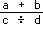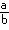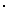﻿ Conventions Governing Order of Evaluation

Conventions Governing Order of Evaluation
Kenneth E. Iverson

The common conventions for the evaluation of unparenthesized expressions include the rules that (1) in a multilevel expression such as, each line is evaluated before the function connecting the lines is evaluated; (2) subject to the first rule, multiplication and division are performed before addition and subtraction; (3) subject to the first two rules, evaluation proceeds from left to right; (4) division can be represented by three distinct but synonymous symbols (a÷b , a/b , and); and (5) multiplication can be represented by two distinct but synonymous symbols (a×b and ab ), or the symbol can be elided. The one convention used in this book is that (subject to parentheses) evaluation proceeds from right to left. This appendix treats the major reasons for this choice.

The common conventions are usually defended on the grounds that they are simple and well known and that their use significantly simplifies the reading and writing of expressions. Because of the familiarity of certain common constructions, these conventions appear simple, but this simplicity is illusory and vanishes on closer examination. Inquiries among students and colleagues have shown such disagreement on the interpretation of the conventions as to dispel the notion that they are well known. Finally, the much simpler convention adopted in this text proves at least as effective in simplifying the reading and writing of expressions.

Consider, for example, the expressions x÷y×z and x÷yz . According to the rules, both are equivalent to the expression (x÷y)×z . However, yz is frequently used as an expression for multiplication which is performed first regardless of other rules. Furthermore, the dot notation for multiplication yields the expression x÷yz , which (according to the interpretations encountered) seems to fall midway between the other cases. Proponents of the common convention protest that such expressions would be parenthesized anyway for clarity; but then the convention seems to lose most of its value.

Matters are further complicated by the alternative notations for division. For example x÷y÷z and x÷y/z should have the same interpretation, but frequently they do not. Similarly, the formally equivalent expressions x+a÷y+b and x+a/y+b frequently receive different interpretations. It is interesting to consider the different possible evaluations of the following expressions which, according to rule 3, are equivalent:

 x÷y×z x÷yz x÷yz x/y×z x/yz x/yz

The common convention also appears to include a number of tacit rules that writers obey automatically. For example, xy may be written for x×y , and any variable should be replaceable by a numerical value. However, while the expression 3y is commonplace, most readers would find the expressions x3 and 3 4 jarring and perhaps inadmissible as expressions for x×3 and 3×4 .

In spite of these defects, the common conventions are reasonably convenient when applied to simple expressions involving only the four basic arithmetic functions, but more serious difficulties arise in their haphazard extension to other functions. For example, the expression sin n × cos m would be interpreted as (sin n)×(cos m) , whereas sin n × π would be interpreted as sin(n × π) . Moreover, the expression abcd is usually interpreted as a(b(cd)) rather than as ((ab)c)d (that is, from right to left rather than from left to right according to rule 3), apparently because the latter case can be expressed by the equivalent expression ab×c×d . In the notation used in this book the first case would be expressed as either a*b*c*d or */a,b,c,d and the second as either a*b×c×d or a*×/b,c,d .

As further functions are introduced (for example, absolute value, maximum, minimum, residue, the relations, logical functions, and the circular functions), the complexity grows and the utility of any relative priority of execution among the functions decreases. Mathematical texts handle this problem either by liberal use of parentheses or by ad hoc (and frequently unstated) conventions. Programming languages, which must face the issue more formally, have usually treated the problem by establishing a hierarchy of priorities among the functions such that any function is evaluated before all others having lower priorities. Such a system is usually very complex (Algol, one of the best known, has nine priority levels) and can therefore be used efficiently only by a programmer who employs it frequently. The occasional (and the prudent) programmer avoids the whole issue by including all the parentheses that would have been required with no convention.

Further examples of the complexity and ambiguity of the common conventions could be easily adduced. However, the skeptical reader will find it more instructive to scan various textbooks trying to formulate precisely the rules used (stated or implied) and applying them rigorously.

The question of the efficacy of the common convention in reducing the need for parentheses will now be addressed. Any convention will reduce the need for parentheses, but the important question is how the common convention compares in this respect with other conventions, and in particular with the notation used in this text.

The utility of the common convention stands forth well in the expression for a polynomial. For example, in the expression

```   axp + bxq + cxr
```

it would be awkward to have to enclose each term in parentheses. However, in the present notation this would be written as

```   +/(a,b,c)×x*p,q,r
```

or, if the vectors of coefficients and exponents were denoted by c and e respectively, then it would be written as

```   +/c×x*e
```

These forms make clear the structure of the polynomial while permitting suppression of detail by using vectors; the corresponding expression in conventional notation is

```   c1×xe1 + c2×xe2 + ... +cn×xen ,
```

where n is the magic variable that denotes the dimensions of all vectors.

The expression (derived in Chapter 4) for the efficient evaluation of a polynomial such as (a,b,c,d,e,f)Πx provides a further example. In the notation used in this text it appears (without parentheses) as

```   (a,b,c,d,e,f)Πx ≡ a+x×b+x×c+x×d+x×e+x×f
```

whereas in the common convention it would appear as

```   (a,b,c,d,e,f)Πx ≡ a+x×(b+x×(c+x×(d+x×(e+x×f))))
```

Further examples could be adduced, but again the skeptical reader will find it more instructive to formulate a set of precise rules based on the common convention and to translate into the resulting notation the expressions appearing in the present text.

There is one further argument against imposing a priority among functions in the present notation. If F and G are dyadic functions, then the expression F/x G y would have either of two interpretations (that is, (F/x)G y or F/(x G y)), depending upon the relative priorities of F and G . These two interpretations differ markedly in form and would therefore lead to confusion. For example, +/x×y would be interpreted as +/(x×y) whereas the similar expression ×/x+y would be interpreted as (×/x)+y . Similar remarks apply to the matrix product M F.G N (defined in Chapter 9).

The reasons for choosing a right-to-left instead of a left-to-right convention are:

 1 The usual mathematical convention of placing a monadic function to the left of its argument leads to a right-to-left execution for monadic functions; for example, F G x ≡ F (G x) . 2 The notation F/z for reduction (by any dyadic function F) tends to require fewer parentheses with a right-to-left convention. For example, expressions such as +/(x×y) or +/(u/x) tend to occur more frequently than (+/x)×y and (+/u)/x . 3 An expression evaluated from right to left is the easiest to read from left to right. For example, the expression ``` a+x×b+x×c+x×d+x×e+x×f ``` (for the efficient evaluation of a polynomial) is read as a plus the entire expression following, or as a plus x times the following expression, or as a plus x times b plus the following expression, and so on. 4 In the definition ``` F/x ≡ x1 F x2 F x3 F ... F x⍴x ``` the right-to-left convention leads to a more useful definition for nonassociative functions F than does the left-to-right convention. For example, -/x denotes the alternating sum of the components of x , whereas in a left-to-right convention it would denote the first component minus the sum of the remaining components. Thus if d is the vector of decimal digits representing the number n , then the value of the expression 0=9|+/d determines the divisibility of n by 9 ; in the right-to-left convention, the similar expression 0=11|-/d determines divisibility by 11 .

Originally appeared as Appendix A of K.E. Iverson, Elementary Functions: An Algorithmic Treatment, Science Research Associates, 1966.

 created: 2009-09-09 13:35 updated: 2013-07-23 09:35